1216.0.55.004 - Information Paper: Converting Data to the Australian Statistical Geography Standard, 2012
Latest ISSUE Released at 11:30 AM (CANBERRA TIME) 28/02/2012  First Issue
Page tools:Print AllRSSSearch this Product

GEOGRAPHIC CORRESPONDENCES

Where other options for converting data are not available, a correspondence can be generated, enabling users to convert data to the ASGS. Correspondence tables detail a mathematical transformation that can be utilised to convert data from one geographic area onto another, unrelated, area. There are many issues that arise with the use of correspondences however, and users need to be aware of these issues and exercise caution when using them to convert data.

Correspondence tables specify the proportion of data for an area that should be donated to another area, effectively converting the geographic base of the data. The area that is donating is known as the "From" unit, and data is allocated to a "To" unit. Examples of the results from a generated correspondence are shown below.

 Table 3: Example of SLA to SA2 Correspondence File FROM UNIT: SLA CODE FROM UNIT: SLA NAME TO UNIT: SA2 CODE TO UNIT: SA2 NAME RATIO OF SLA WITHIN SA2 PERCENT OF SLA WITHIN SA2 105051100 Botany Bay (C) 117011320 Banksmeadow 0.0009346 0.09346 105051100 Botany Bay (C) 117011321 Botany 0.2219453 22.19453 105051100 Botany Bay (C) 117011322 Mascot - Eastlakes 0.491931 49.1931 105051100 Botany Bay (C) 117011323 Pagewood - Hillsdale - Daceyville 0.2851891 28.51891 105054800 Leichhardt (A) 120021387 Balmain 0.3055177 30.55177 105054800 Leichhardt (A) 120021388 Leichhardt - Annandale 0.4496677 44.96677 105054800 Leichhardt (A) 120021389 Lilyfield - Rozelle 0.2448146 24.48146

As can be seen from Table 3, the first two columns contain Statistical Local Area (SLA) codes and SLA names. In this correspondence SLAs represent the "From" units, or the units that data is being converted from. The SA2s represent the "To" units, or the units that data is being converted to. Ratio details the proportion of data that the "From" unit is donating to the respective "To" units.

In this example the SLA of Botany Bay is divided across four separate SA2s. 0.09% of the data for the SLA of Botany Bay is allocated to the SA2 of Banksmeadow, and 22.19% of the data for the SLA of Botany Bay is allocated to the SA2 of Botany. 49.19% is allocated to Mascot - Eastlakes and 28.52% to Pagewood - Hillsdale - Daceyville. The correspondence is then applied to a data value for each donating area, and the aggregate data for each "To" area calculated.

Weighting of Correspondences

When correspondences are created the ABS uses a weighting unit to assist with the allocation of "From" data to their respective "To" units. The unit that is used to weight the correspondence impacts on how the "From" units are apportioned, which in turn can have a major impact on the data values and hence the quality of the converted, or corresponded, data.

The unit used to weight a correspondence can vary, depending on the intended use and the nature of the data being converted. For example, correspondences can be weighted by area, by Mesh Block dwelling or population counts, or by particular population characteristics. The weighting unit is most effective when it is smaller than the geographic units being converted. Research and testing has also shown that the relationship between the weighting unit and the data being converted is critical. For instance, if agricultural or environmental data is being converted then using area as the weighting unit is quite effective as it assumes an even distribution of data, and these types of data tend to be uniform. However, using area as the weighting unit when converting other types of data, such as population-based data, can lead to poor results.

An example to consider is a case where there is a requirement to convert data from SLA to SA2 and one SLA encompasses two SA2s of equal size. One of the SA2s contains nursing homes whereas the other is made up of an industrial estate which contained no population on Census night. If an area weighted correspondence was used the population of the SLA would be evenly distributed between the SA2s. Given that only one of the SA2s contains population, distributing the population using this method will lead to incorrect and misleading results. However if a Census population weighted correspondence was used, the SA2 that contains the nursing homes would be allocated the entire population, and the SA2 containing the industrial estate would receive a zero allocation of population. This would result in an accurately distributed population and would reflect the true characteristics of the two SA2s.

A further example shows the comparison of actual and converted Deaths data using different SLA to SA2 correspondences. Figure 2 represents data converted by an area weighted correspondence whereas the data in Figure 3 has been weighted using Collection District (CD) counts of persons aged 65 and over from the 2006 Census.

Each point represents the actual data value plotted against its converted value for a given geographic area in the correspondence table. For example, in Figure 2 the highest point plotted for converted deaths is 292 whereas the actual deaths figure for the SA2 in question is 7. This indicates that the data transformation for that area was not accurate. A perfect correspondence would be represented by a plot showing a straight line rising at an angle representing a 1 to 1 ratio.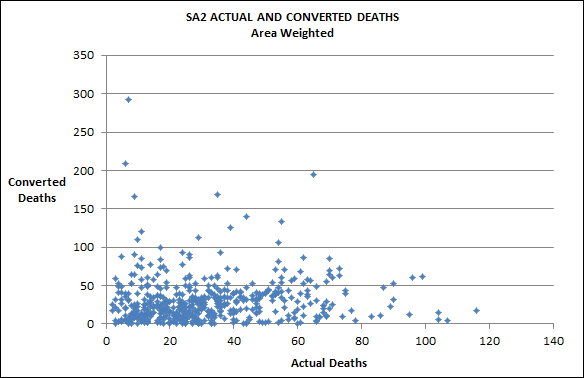Figure 2: CORRESPONDED DEATHS DATA - Area weighted.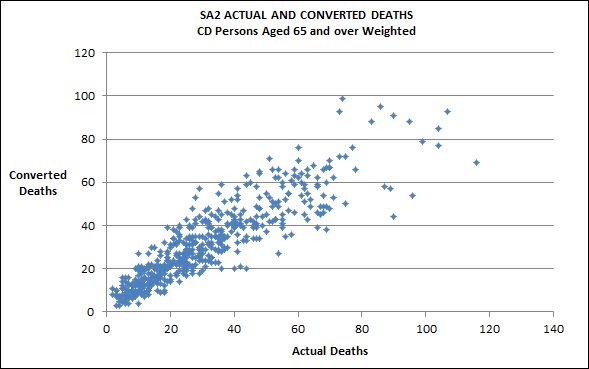Figure 3: CORRESPONDED DEATHS DATA - Weighted by Persons aged 65 and over.

It can be clearly seen in this instance that the area based correspondence has produced a markedly inferior result when compared to the population weighted data.

As can be seen in Figure 2, there are many points where the actual and converted counts are not similar at all, so the conclusion can be made that using an area weighted correspondence to convert this data is not suitable. However, in Figure 3, where the weighting unit is CD counts of persons aged 65 years and over, the actual and converted counts are more similar and show a better, though not perfect, result. The conclusion here is that this correspondence is converting data to a higher degree of accuracy. This is not surprising, given that the data is population-based, and is being converted using a population weighted correspondence.

Figures 2 and 3 showed examples of data being converted to differing degrees of accuracy. To contrast this is an example where data is being converted to a high level of accuracy. Figure 4 shows the results of geocoded address points being converted from SLA to Statistical Area Level 3 (SA3) where estimated dwellings at the Mesh Block level have been used as the weighting unit.

Figure 4 highlights two issues. Firstly, that converting smaller geographic units to larger units will generally result in more accurate data conversion than will be returned when converting areas of similar size, or when converting larger areas to smaller areas. In this example converting SLA level data to represent larger SA3 level data produces excellent results as SLAs are a smaller geographic unit than SA3s. The second issue demonstrated in Figure 4 is the importance of the weighting unit when converting data. The weighting unit used with this correspondence was estimated dwellings at the Mesh Block level. As dwelling estimates relate closely to the G-NAF, that is the data source, this correspondence was ideal for converting G-NAF counts from SLA to SA3.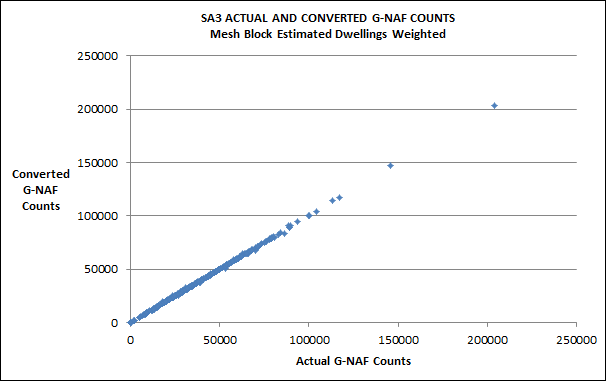Figure 4: CORRESPONDED G-NAF COUNTS - SLA to SA3.

Another issue that needs to be considered is that the same correspondence will convert different types of data to differing degrees of accuracy. This is highlighted in Figures 5 and 6.

Figure 5 details the results of converting G-NAF counts from SLA to SA2 where estimated dwellings at Mesh Block level is used as the weighting unit, whereas Figure 6 shows the results of converting deaths data from SLA to SA2 using the same weighting unit. In this instance the same correspondence is being used to convert different types of data, and as can be seen by the results, the G-NAF counts have been converted to a higher degree of accuracy than the Deaths data. The reason in this case is that the weighting unit being used, Mesh Block estimated dwellings, relates more closely to G-NAF data than Deaths data. This highlights the fact that the relationship between the data to be converted and the weighting unit used in the correspondence is critical to the accuracy of the output data.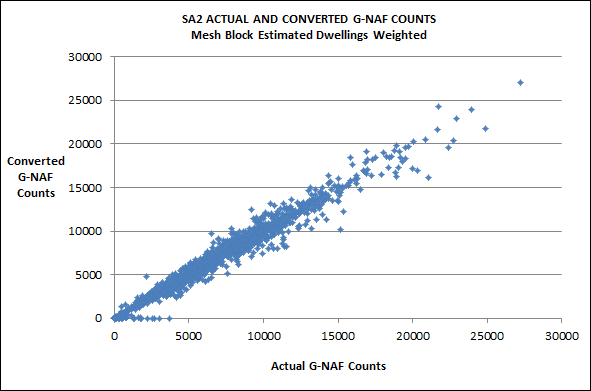Figure 5: CORRESPONDED G-NAF DATA - Weighted by estimated dwelling counts.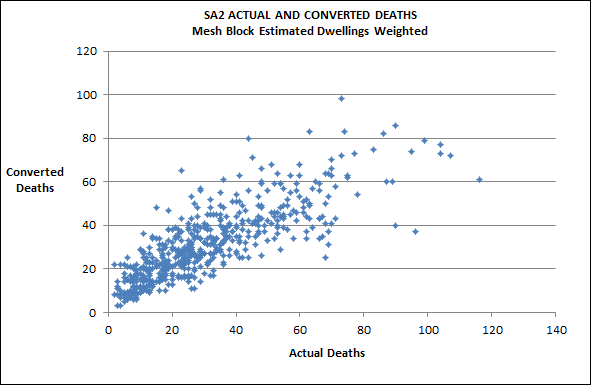Figure 6: CORRESPONDED DEATHS DATA - Weighted by estimated dwelling counts.